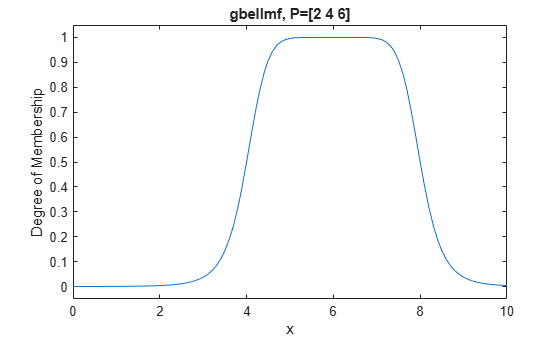gbellmf

Generalized bell-shaped membership function

Description

This function computes fuzzy membership values using a generalized bell-shaped membership function. You can also compute this membership function using a fismf object. For more information, see fismf Object.

example

y = gbellmf(x,params) returns fuzzy membership values computed using the following generalized bell-shaped membership function:

$f\left(x;a,b,c\right)=\frac{1}{1+{|\frac{x-c}{a}|}^{2b}}$

To configure the membership function, specify parameters, a, b, and c using params.

Membership values are computed for each input value in x.

Examples

collapse all

x = 0:0.1:10;
y = gbellmf(x,[2 4 6]);
plot(x,y)
xlabel('gbellmf, P=[2 4 6]')Input Arguments

collapse all

Input values for which to compute membership values, specified as a scalar or vector.

Membership function parameters, specified as the vector [a b c].

Here:

• a defines the width of the membership function, where a larger value creates a wider membership function.

• b defines the shape of the curve on either side of the central plateau, where a larger value creates a more steep transition.

• c defines the center of the membership function.

Output Arguments

collapse all

Membership value returned as a scalar or a vector. The dimensions of y match the dimensions of x. Each element of y is the membership value computed for the corresponding element of x.

Alternative Functionality

fismf Object

You can create and evaluate a fismf object that implements the gbellmf membership function.

mf = fismf("gbellmf",P);
Y = evalmf(mf,X);

Here, X, P, and Y correspond to the x, params, and y arguments of gbellmf, respectively.